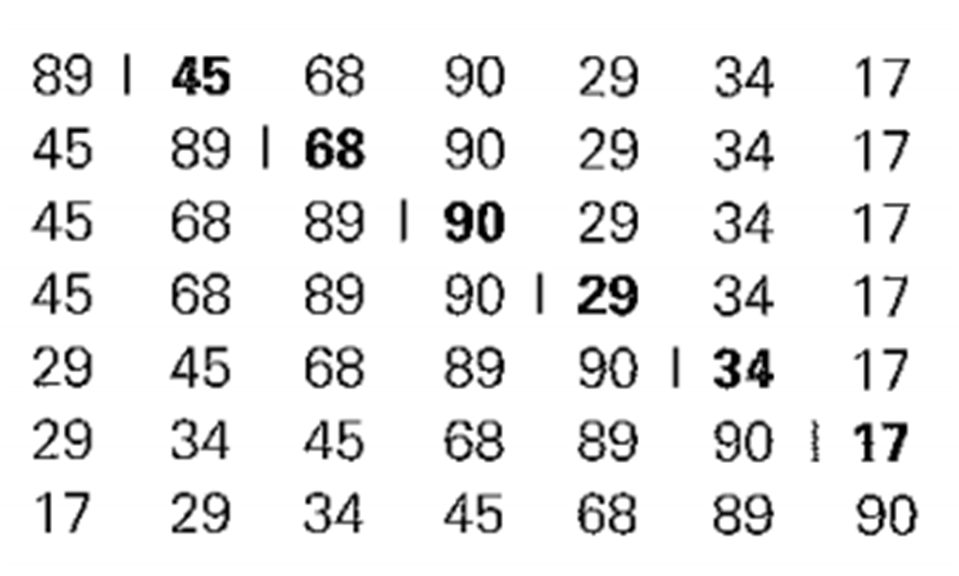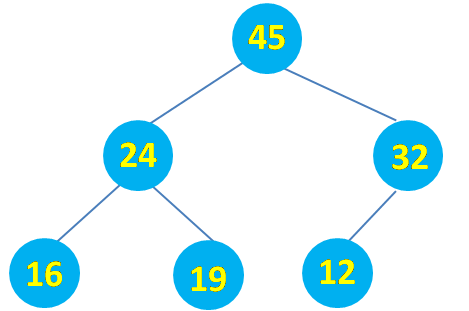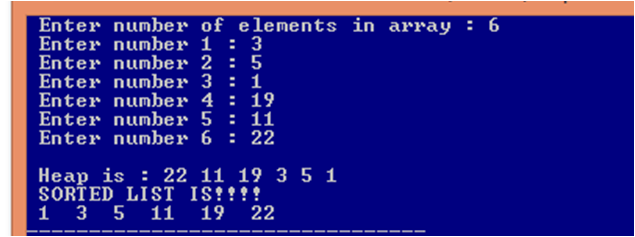## Program to find whether a given number is Prime or Not

```#include<stdio.h>

int main()
{
/* Declaring variable for n=number, c=counter variable:holds
number of factor of 'n' */
int n,c=0, i;

// Inputting number
printf("Enter number to check whether Prime or Not:");
scanf("%d",&n);

// Checking whether number valid or not
if(n>0)
{

// Checking whether number is prime or not
for(i=1;i<=n;i++)
{
if(n%i==0)
c=c+1;
}

if(c==2)
printf("Prime Number");
else
printf("Not a Prime Number");
}

else
printf("Not a valid Number");```
```
return 0;
}
```

## Program to print ( list ) all the prime number between 1 to n

```#include<stdio.h>

int main()
{
/* Declaring variable for n=number, c=counter variable:holds
number of factor of 'n' */
int n,c, i,j;

// Inputting number
printf("Enter number till which you want to list prime number:");
scanf("%d",&n);

// Checking whether number valid or not

if(n>0)
{
printf("List of Prime Numbers:");
// Checking whether number is prime or not
for(i=1;i<=n;i++)
{
c=0;
for(j=1;j<=i;j++)
{
if(i%j==0)
c=c+1;
}
if(c==2)
printf("%d ",i);
}

}
else
printf("Not a valid Number");

return 0;
}```

## Palindrome Program In C

C PROGRAM TO DETERMINE PALINDROME NUMBER

Back To Palindrome Program

```#include<stdio.h>
int main()
{
// variable for a=number, n=to copy number, d=digit, rev=reverse of number
int a,n,d=0,rev=0;

// Inputting number
printf("Enter any number to determine whether it is Palindrome or not:");
scanf("%d",&a);

// copying number in n
n=a;

// Determining whether number is palindrome or not
while(n!=0)
{
d=n%10;
rev=rev*10+d;
n=n/10;
}

// Displaying Whether number is palindrome or not
if(a==rev)
printf("Palindrome number");
else
printf("Not a Palindrome Number");

return 0;
}
```

C PROGRAM TO PRINT(LIST) PALINDROME NUMBER FROM 10 TO n

Back To Palindrome Program

`#include<stdio.h>`
```int main()
{
/*declaring variable for num=user wish, i=acts as number, n=to copy number,
d=digit, rev=reverse of number */
int num,n,d=0,rev,i;

// Inputting number
printf("Enter number till which you want to list Palindrome Number:");
scanf("%d",&num);

printf("List of Palindrome Number:");
for(i=10;i<=num;i++)
{
// copying number in n
n=i;
rev=0;
// Determining whether number is palindrome or not
while(n!=0)
{
d=n%10;
rev=rev*10+d;
n=n/10```
```    }

// Displaying Whether number is palindrome or not
if(i==rev)
printf("%d ",i);
}

return 0;```
```}
```

C PROGRAM TO FIND WHETHER A NUMBER IS PALINDROME OR NOT USING RECURSION

Back To Palindrome Program

```#include<stdio.h>

// Declaring global variable r=reverse, d=digit
int r=0, d=0;

// Defining function with parameter n = number
int rev(int n)
{
/* Base condition : Any condition where a recursive function
or method does not invoke itself. */
if(n==0)
return r;

// Continue calling function rev or function invoke itself
else
{
// Extracting digit
d=n%10;

// Finding reverse
r=(r*10+d);

// function invoke itself
rev(n/10);
}
}

int main()
{
// Declaring variable n = number
int n;

// Declaring variable "r" to hold the reverse number
int r;

// Inputting Number
printf("Enter the Number : ");
scanf("%d",&n);

// Calling function "rev" with actual parameter "n" passed to it
r=rev(n);

// Checking and Displaying if a Number is palindrome or Not
if(r==n)
printf("%d is a Palindrome Number ",n);

else
printf("%d is not a Palindrome Number ",n);```
```
return 0;
}
```

C PROGRAM TO ENTER A WORD AND CHECK WHETHER IT IS PALINDROME OR NOT USING STRING FUNCTION

Back To Palindrome Program

```// palindrom - When word = reverse of word then it is called palindrome

#include<stdio.h>
#include<string.h>

int main()
{
// Declaring variable str=string
char word, word1;

// Inputing string
printf("Enter any word : ");
scanf("%s",&word);

// Copying word to word1
strcpy(word1,word);

// checking if palindrome or not
if(strcmp(word,strrev(word1))==0)
{
printf("Entered word is a palindrome ");
}

else
printf("Entered word is not palindrome ");

return 0;
}
```

PALINDROME PROGRAM IN C WITHOUT USING STRING FUNCTION

Back To Palindrome Program

```#include<stdio.h>

int main()
{
// Declaring variable str=string
char word, revword;

/* Declaring variable i=to iterate loop, l=length,
c=count the number of matched character */
int i, j, l=0, c;

// Inputing string
printf("Enter any word : ");
scanf("%s",&word);

// finding length
while(word[l]!='\0')
l++;

// Reversing string
j=0;
for(i=l-1;i>=0;i--)
{
revword[j]=word[i];
j++;
}
revword[j]='\0';

//checking if palindrome or not
c=0;
for(i=0;word[i]!='\0';i++)
{
if(revword[i]==word[i])
c++;
}

if(c==l)
{
printf("Word is a palindrome ");
}

else
printf("Word is not palindrome ");

return 0;
}
```

## Leap Year Program In C

### Leap Year Program In C using if-else

// Leap year program in c using if-else
```#include<stdio.h>

int main()
{
// Declaring variable for y=year
int y;

// Inputing year
printf("Enter year to check leap year or not: ");
scanf("%d",&y);

// Determining and displaying whether leap year or not
if(y%4==0)
{
if(y%100==0 && y%400!=0)
printf("Century year, But not a leap year");
else
printf("Leap year");
}
else
printf("Not a Leap Year");

return 0;
}
```

### Leap Year Program In C Using Ternary

// Leap year program in c using ternary
```#include<stdio.h>

int main()
{
// Declaring variable "y" to input year and "leap" to determine leap year
int y,leap;

// Inputting year
printf("Enter any year : ");
scanf("%d",&y);

// Checking if leaf year or not
leap=(y%400==0)?:(y%100!=0)?(y%4==0)?1:0:0;

if(leap==1)
printf("The given year is leap year");
else
printf("The given year is not leap year");

return 0;
}
```

## INSERTION SORT IN C

In Insertion Sort we select a key i.e an element one by one from any given list of element ( array ) and then we insert it in its appropriate position. We can either scan the list from left to right or right to left to find an appropriate position. But usually we scan list from right to left because it is better in case of sorted and almost sorted arrays. Insertion sort is an efficient algorithm for sorting small number of elements. Though Insertion Sort is based on recursive idea, it is more efficient to implement this algorithm by bottom up approach i.e iteratively.

Need Programming Help visit: Get Programming Help Online

Consider the elements to be sorted by insertion sort are : 89, 45, 68, 90, 29, 34, 17

As shown in Figure below, starting with A and ending with A[n - 1], A[i] is inserted in its appropriate place among the first i elements of the array that have been already sorted (but, unlike selection sort, the elements are generally not in their final positions).Insertion Sort In C : Showing how elements are sorted
The above figure shows how elements 89, 45, 68, 90, 29, 34, 17  are sorted by insertion sort. A vertical bar separates the sorted part of the array from the remaining elements; the element being inserted is in bold.

Note : Refer to algorithm for better understanding.

## INSERTION SORT ALGORITHM

```InsertionSort(int a[ ], int n)

for i=1 to n-1
key = a[i]
j = i-1```
```
while j>=0 and key < a[j]
a[j+1]=a[j];
j=j-1;

a[j+1] =  key
```

Note : The operation of the algorithm can be understood with the help of above figure.

## TIME COMPLEXITY OF INSERTION SORT

### Time Complexity Of Insertion Sort - Best Case

• The best case input in an array is such that the array is already sorted.
• In this case insertion sort has linear running time i.e O(n)

### Time Complexity Of Insertion Sort -Worst Case

• The worst case input in an array is such that the array is sorted in reverse order.
• In this case insertion sort has quadratic running time i.e O(n2

### Time Complexity Of Insertion Sort -Average Case

• Input in this case is a random input.
• In this case also insertion sort has quadratic running time i.e O(n2)
• Insertion Sort is very efficient in sorting very small arrays.
• Its impractical to sort very large arrays using insertion sort due to its time complexity of O(n2)

## INSERTION SORT PROGRAM IN C

```// Insertion sort program in c

#include<stdio.h>

void InsertionSort(int a[],int n) ;

int main()
{
int a,i,n;
printf("Enter number of elements in array : ");
scanf("%d",&n);

// Inputting elements
for(i=0;i<n;i++)
{
printf("Enter number %d: ",i+1);
scanf("%d",&a[i]);
}

// Displaying Elements before insertion sort
printf("Items in the array are : ");
for(i=0;i<n;i++)
{
printf("%d ",a[i]);
}

//Applying insertion sort
InsertionSort(a,n);

// Displaying elements after insertion sort
printf("\nElements after insertion sort : ");
for(i=0;i<n;i++)
{
printf("%d ",a[i]);
}
return 0;
}

void InsertionSort(int a[],int n)
{
int i,key,j;

for(i=1;i<n;i++)
{
key=a[i];
j=i-1; ```
```
```
```  // Finding appropriate position to insert key
while((key<a[j])&&(j>=0))
{
a[j+1]=a[j];
j=j-1;
}
```
```  // Inserting key
a[j+1]=key;
}
}

```

## Pattern Programs In C

1. Program to print the given pattern :
*
* *
* * *
* * * *
* * * * *
2. Program to print the given pattern :
*
* *
* * *
* * * *
* * * * *
* * * * * * ..... till n rows
3. Program to print the given pattern :
1
1 2
1 2 3
1 2 3 4
1 2 3 4 5 .... till n rows
4. Program to print the given pattern :
1
2 2
3 3 3
4 4 4 4
5 5 5 5 5 .... till n rows
5. Program to print the given pattern :
1 1 1 1 1
2 2 2 2 2
3 3 3 3 3
4 4 4 4 4
5 5 5 5 5
6. Program to print the given pattern :
5
5 4
5 4 3
5 4 3 2
5 4 3 2 1
7. Program to print the given pattern :
1 2 3 4 5
1 2 3 4
1 2 3
1 2
1
8. Program to print the given pattern :
5 4 3 2 1
5 4 3 2
5 4 3
5 4
5
9. Program to print the given pattern :
```        *
* *
* * *
* * * *
* * * * *
```
10. Program to print the given pattern :
```        1
1 2
1 2 3
1 2 3 4
1 2 3 4 5```
11. Program to print the given pattern :
```        5
4 5
3 4 5
2 3 4 5
1 2 3 4 5
```
12. Program to print the given pattern :
1
* *
1 2 3
* * * *
1 2 3 4 5 .... till n rows
13. Program to print the given pattern :
```1                 1
1 2             1 2
1 2 3         1 2 3
1 2 3 4     1 2 3 4
1 2 3 4 5 1 2 3 4 5
```
14. Program to print the given pattern :
```1                 1
1 2             2 1
1 2 3         3 2 1
1 2 3 4     4 3 2 1
1 2 3 4 5 5 4 3 2 1
```
15. Program to print the given pattern :
```1                 5
1 2             5 4
1 2 3         5 4 3
1 2 3 4     5 4 3 2
1 2 3 4 5 5 4 3 2 1
```
16. Program to print the given pattern :
1
2 6
3 7 10
4 8 11 13
5 9 12 14 15 ... till n rows
17. Program to print the given pattern :
```******** ********
*******   *******
******     ******
*****       *****
****         ****
***           ***
**             **
*               *

*               *
**             **
***           ***
****         ****
*****       *****
******     ******
*******   *******
******** ********
```
18. Program to print the given pattern
```
*
***
*****
*******
*********
*******
*****
***
*
```
19. Program to print the given pattern :
8 4 2 1
4 2 1
2 1
1
20. Program to print Pascal triangle :

## HEAP SORT IN C

Heap sort uses a binary heap, which is a complete binary tree.

Binary Tree : A Binary Tree is a hierarchical structure  that is either empty or consists of an element, called the root, and two distinct binary trees, called the left subtree and right subtree.

Example :Heap Sort : Binary Tree

Heap is a binary tree with the following properties :
• It is a complete binary tree.
•  Each node is greater than or equal to any of its children.
Complete Binary Tree : A binary tree is complete if each of its levels is full, except that the last level may not be full and all the leaves on the last level are placed leftmost.

Example :Heap Sort : Complete Binary Tree

Heap Example :Heap Sort : Heap

Note : We represent heaps in level order, going from left to right. The array corresponding to the heap above is  :
[45, 24, 32, 16,19,12, 21].

## PSEUDOCODE TO ADD NODE IN HEAP

To add a new node to the heap, first add it to the end of the heap and then rebuild the tree as follows:

Let the last node be the current node;
while (the current node is greater than its parent) {
Swap the current node with its parent;
Now the current node is one level up;
}

Suppose we want to insert 3, 5, 1, 19, 11, 22. We assume that the heap is initially empty.Heap Sort : Adding Node In Heap
Now lets insert a new node 88 in the above heap.Heap Sort : Rebuilding of Heap after adding a new node

## PSEUDOCODE TO REMOVE ROOT FROM HEAP

Often you need to remove the max element, which is the root in a heap. After the root is removed, the tree must be rebuilt to maintain the heap property. The algorithm for rebuilding the tree can be described as follows:

Move the last node to replace the root;
Let the root be the current node;
while (the current node has children and the current node is smaller than one of its children) {
Swap the current node with the larger of its children;
Now the current node is one level down;
}Heap Sort : Removing Root and Rebuilding Heap

## HOW HEAP SORT IS PERFORMED

• First we take input in an array.
• Then we insert these element in heap.
• As we know that root of the heap is maximum element so we remove root and rebuild heap so that the next maximum element is at root. Rebuilding ensures that our tree is in the form of heap.
• We store the root ( maximum element ) in decreasing order of array length. We could have used another array as well to store the root.

## HEAP SORT PROGRAM IN C

`// C Program ( Code ) for Heap sort`
```#include<stdio.h>

void insert(int a[], int n, int item);
int deleteheap(int a[],int n);

int main()
{
int a,i,n,item;
printf(" Enter number of elements in array : ");
scanf("%d",&n);
for(i=0;i<n;i++)
{
printf(" Enter number %d : ",i+1);
scanf("%d",&a[i]);
}

for(i=0;i<n;i++)
{

item=a[i];
insert(a,i,item);
}
printf("\n Heap is : ");
for(i=0;i<n;i++)
{
printf("%d ",a[i]);
}

for(i=n;i>=1;i--)
{
item=deleteheap(a,i);

// Stores item=Maximum element in decreasing order of array length
a[i-1]=item;
}

printf("\n SORTED LIST IS!!!!\n");
for(i=0;i<n;i++)
{
printf(" %d ",a[i]);
}

}

// Used to Build Heap
void insert(int a[], int n, int item)
{
int ptr,par,temp;

ptr=n;
while(ptr>=1)
{
par=(ptr-1)/2;

if(a[par]>=item)
{
a[ptr]=item;
return;
}
else
{
a[ptr]=a[par];
ptr=par;
}

a[ptr]=item;
}
}

/* Remove root which is the maximum element in Heap and rebuild heap
so that the next maximum element is at root. Rebuilding ensures
that our tree is in the form of heap.
Returns item = a wcich contains the root of the heap.
*/
int deleteheap(int a[],int n)
{
int i=0,item,temp;

// a - contains the root of the heap
item=a;
a=a[n-1];
n=n-1;
while(((a[i]<a[2*i+1])||(a[i]<a[2*i+2]))&&((2*i+1)<n))
{
if(a[2*i+1]>a[2*i+2])
{
temp=a[i];
a[i]=a[2*i+1];
a[2*i+1]=temp;
i=2*i+1;
}

else
{
temp=a[i];
a[i]=a[2*i+2];
a[2*i+2]=temp;
i=2*i+2;
}
}

// Root of the heap is returned
return item;
}
```

## HEAP SORT PROGRAM OUTPUTHeap Sort Output